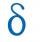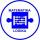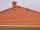# Third member

Determine the third member of the AP if a4=93, d=7.5.

Result

a3 =  85.5

#### Solution:Leave us a comment of example and its solution (i.e. if it is still somewhat unclear...):

Showing 0 comments:Be the first to comment!## Next similar examples:

1. Fifth memberDetermine the fifth member of the arithmetic progression, if the sum of the second and fifth members equal to 73, and difference d = 7.
2. AP - basicsDetermine first member and differentiate of the the following sequence: a3-a5=24 a4-2a5=61
3. Difference APCalculate the difference of arithmetic progression if the sum of its first 19 members Sn = 8075 and the first member is a1 = 20
4. GP membersThe geometric sequence has 10 members. The last two members are 2 and -1. Which member is -1/16?
5. AS sequenceIn an arithmetic sequence is given the difference d = -3 and a71 = 455. a) Determine the value of a62 b) Determine the sum of 71 members.
6. AP - simpleDetermine the first nine elements of sequence if a10 = -1 and d = 4
7. SequenceBetween numbers 1 and 53 insert n members of the arithmetic sequence that its sum is 702.
8. Sequence 3Write the first 5 members of an arithmetic sequence: a4=-35, a11=-105.
9. SequenceWrite the first 6 members of these sequence: a1 = 5 a2 = 7 an+2 = an+1 +2 an
10. AS - sequenceWhat are the first ten members of the sequence if a11=22, d=2.
11. Sequence 2Write the first 5 members of an arithmetic sequence a11=-14, d=-1
12. SequenceWrite the first 7 members of an arithmetic sequence: a1=-3, d=6.
13. Difference AP 4Calculate the difference of the AP if a1 = 0.5, a2 + a3 = -1.1
14. SeatsSeats in the sport hall are organized so that each subsequent row has five more seats. First has 10 seats. How many seats are: a) in the eighth row b) in the eighteenth row
15. RoofTiles are stacked in rows on the trapezoidal shaped roof. At the ridge is 15 tiles and each subsequent row has one more tile than in the previous row. How many tiled is covered roof if lowermost row has 37 tiles?
16. Parametric equationFind the parametric equation of a line with y-intercept (0,-4) and a slope of -2.
17. Theorem proveWe want to prove the sentense: If the natural number n is divisible by six, then n is divisible by three. From what assumption we started?